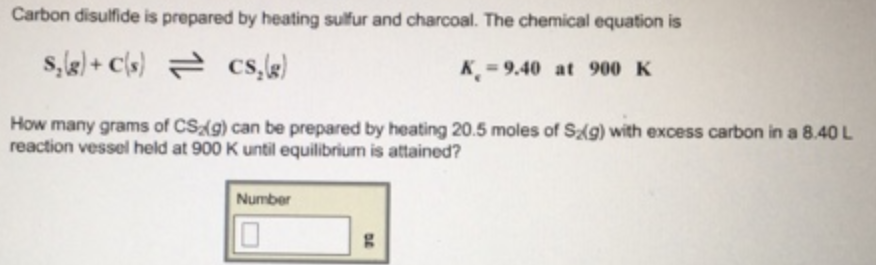# Problem: Carbon disulfide is prepared by heating sulfur and charcoal. The chemical equation isS2 (g) + C (s) ⇌ CS2 (g)      Kc = 9.40 at 900 K How many grams of CS2(g) can be prepared by heating 20.5 moles of S2(g) with excess carbon in a 8.40 L reaction vessel held at 900 K until equilibrium is attained?

###### FREE Expert Solution###### Problem Details

Carbon disulfide is prepared by heating sulfur and charcoal. The chemical equation is

S2 (g) + C (s) ⇌ CS2 (g)      Kc = 9.40 at 900 K

How many grams of CS2(g) can be prepared by heating 20.5 moles of S2(g) with excess carbon in a 8.40 L reaction vessel held at 900 K until equilibrium is attained?